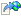﻿CommsMath.CalculateMean Method (List(Double), List(Double))
 CommsMath CalculateMean Method (List Double , List Double ) NetworkComms.Net Help
Return the mean of the provided list of values

Namespace: NetworkCommsDotNet.Tools
Assembly: NetworkCommsDotNet (in NetworkCommsDotNet.dll) Version: 3.0.0.0 (3.0.0.0)
Syntax

```public static double CalculateMean(
List<double> localValues,
List<double> weights
)```

#### Parameters

localValues
Type:System.Collections.Generic ListDouble
Values for which a mean should be calculated
weights
Type:System.Collections.Generic ListDouble
The weights to apply to the corresponding values

#### Return Value

Type:Double
The mean of provided values